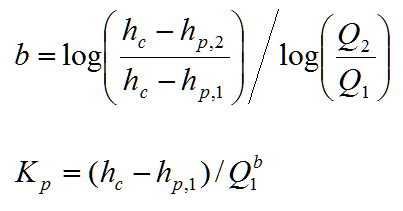AlternateSWMM6

# Pump Characteristic Curve from Innovyze H2OCalc

Pump Characteristic Curve

Pump Characteristic Curve

Pump characteristic curves are usually presented graphically describing the relationship between pump head, hp, and the flow rate, Q using either an exponential equation or 3-point quadratic form. The exponential pump characteristic curve is given aswhere hc = the pump cutoff head associated with the zero flow condition (m, ft)

Kp = resistance coefficient

b = flow exponent

nR = pump speed ratio (the ratio of the actual pump speed to the rated pump

speed); for constant speed pump operation, nR is equal to 1.

Given three hpQ points [hc, (hp,1, Q1), (hp,2, Q2)], the pump curve can be calculated asIf a single rated capacity (hrated, Qrated) is given, two more points can be added to the curve by assuming a shutoff head at zero flow equal to 133% of the design head and a maximum flow at zero head equal to twice the design flow. Then, the curve can be treated as a three-point curve.

The 3-point quadratic type of pump characteristic curve iswhere ap, bp, cp are the coefficients of the quadratic curve.

The coefficients are calculated as